# Three-dimensional shapes

### Term 1 starting in week 7 :: Estimated time: 3 weeks

• Know names of 2-D and 3-D shapes
• Recognise prisms
• Accurate nets of cuboids and other 3-D shapes
• Sketch and recognise nets of cuboids and other 3-D shapes
• Plans and elevations
• Find area of 2-D shapes (review)
• Surface area of cubes and cuboids
• Surface area of triangular prisms
• Surface area of a cylinder
• Volume of cubes and cuboids
• Volume of other 3-D shapes - prisms and cylinders

For higher-attaining pupils:

• Explore volumes of cones, pyramids and spheres

This page should remember your ticks from one visit to the next for a period of time. It does this by using Local Storage so the information is saved only on the computer you are working on right now.

## Lesson Starters

Here are some suggestions for whole-class, projectable resources which can be used at the beginnings of each lesson in this block.

### 1st Lesson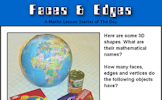#### Faces and Edges

Find the number of faces, edges and vertices on some familiar objects.

### 2nd Lesson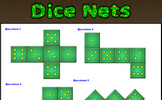#### Dice Nets

Determine whether the given nets would fold to produce a dice.

### 3rd Lesson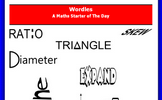#### Wordles

Turn mathematical words into designs which will help you remember the meanings of the words.

### 4th Lesson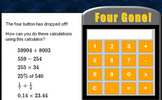#### Four Gone

An activity involving a broken calculator which is missing the four button. Can you evaluate the given expressions without using the four?

### 5th Lesson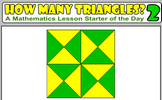#### How Many Triangles? 2

How many triangles are hidden in the pattern? What strategy might you use to count them all to ensure you don't miss any out?

### 6th Lesson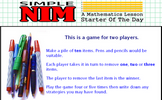#### Simple Nim

The classic game of Nim played with a group of pens and pencils. The game can be extended to the multi-pile version.

### 7th Lesson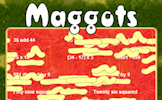#### Maggots

Do as many of the calculations as possible before the maggots infest!

### 8th Lesson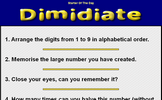#### Dimidiate

Arrange the digits from 1 to 9 in alphabetical order. How many times can this number be halved?

### 9th Lesson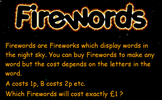#### Firewords

Find words which cost 100p if A costs 1p, B costs 2p etc

Some of the Starters above are to reinforce concepts learnt, others are to introduce new ideas while others are on unrelated topics designed for retrieval practice or and opportunity to develop problem-solving skills.

White Rose ResourcesEnd of block assessments provide a quick progress check at the end of each block of learning to make sure students have understood the content covered. This Scheme of Learning was produced by White Rose Maths and is used here with permission granted on 30th June 2021.For All: